Next: Worked Examples Up: Electric Potential Previous: Electric Potential and Electric

## Electric Potential of a Point Charge

Let us calculate the electric potential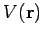generated by a point chargelocated at the origin. It is fairly obvious, by symmetry, and also by looking at Fig. 14, thatis a function ofonly, whereis the radial distance from the origin. Thus, without loss of generality, we can restrict our investigation to the potential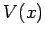generated along the positive-axis. The-component of the electric field generated along this axis takes the form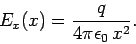(94)

Both the- and-components of the field are zero. According to Eq. (87),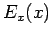andare related via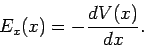(95)

Thus, by integration,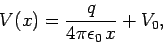(96)

where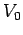is an arbitrary constant. Finally, making use of the fact that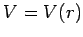, we obtain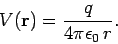(97)

Here, we have adopted the common convention that the potential at infinity is zero. A potential defined according to this convention is called an absolute potential.

Suppose that we havepoint charges distributed in space. Let the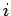th charge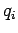be located at position vector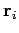. Since electric potential is superposable, and is also a scalar quantity, the absolute potential at position vector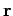is simply the algebraic sum of the potentials generated by each charge taken in isolation: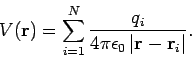(98)

The work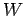we would perform in taking a chargefrom infinity and slowly moving it to pointis the same as the increase in electric potential energy of the charge during its journey [see Eq. (79)]. This, by definition, is equal to the product of the chargeand the increase in the electric potential. This, finally, is the same astimes the absolute potential at point: i.e.,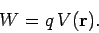(99)Next: Worked Examples Up: Electric Potential Previous: Electric Potential and Electric
Richard Fitzpatrick 2007-07-14